The D Layer Pattern Archive

# The D Layer Pattern Archive

Use the following sequences generate the described pattern from a
solved state, and it's inverse to get back from the pattern to a
solved state.

Note from Cubeman:
I solve the 4 bottom (or D layer) edge cubes last. Here are some of the D layer patterns. All patterns are optimal in q turns. All cube pictures will henceforth be 192x172 pixels so the cubes will look square on 640x480, 800x600 and 1024x768 resolutions.

Special thanks to Mike Reid for providing the optimal solution generator.

The Cube Pattern Archive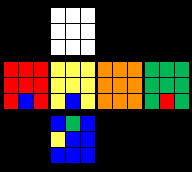C1...F D' F' R L' U F U' R' L...(10f, 10q)
Order = 3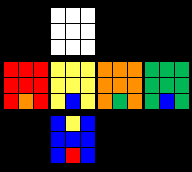C2...F D2 F2 D' R F U F2 U' R' F' D F...(13f, 16q)
Order = 6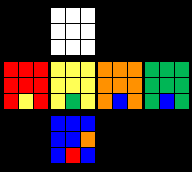C3...R D R' F' U' R2 U R F D' R2 D2 R...(13f, 16q)
Order = 6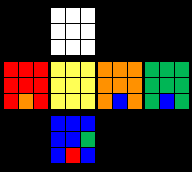C4...B' D2 B R F L D2 L' F' R'...(10f, 12q)
Order = 3C5...B D2 B' L' F' R' D2 R F L...(10f, 12q)
Order = 3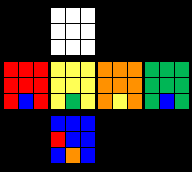C6...L' D' L F U L2 U' L' F' D L2 D2 L'...(13f, 16q)
Order = 6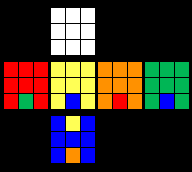C7...F' D2 F2 D L' F' U' F2 U L F D' F'...(13f, 16q)
Order =6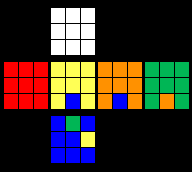C8...F D F' R L' U F' U' R' L...(10f, 10q)
Order = 3C9...F' R F R' L D' R' D R L'...(10f, 10q)
Order = 3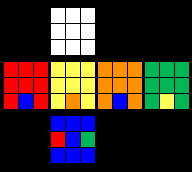C10...L D2 L2 D' F L U L2 U' F' L' D L...(13f, 16q)
Order = 6C11...F L' F' R' L D L D' R L'...(10f, 10q)
Order = 3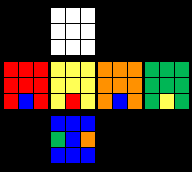C12...R' D2 R2 D F' R' U' R2 U F R D' R'...(13f, 16q)
Order = 6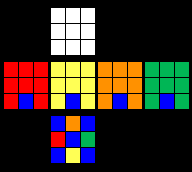C13...B' L' F' D F D' L B R F D F' D' R'...(14f, 14q)
Order = 6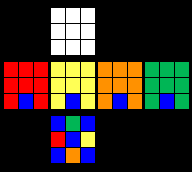C14...F L B D' B' D L' F' R' B' D' B D R...(14f, 14q)
Order = 6

Back to Main